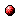# Chance-constrained model predictive controller synthesis for stochastic max-plus linear systems

Reference:
V. Rostampour, D. Adzkiya, S. Esmaeil Zadeh Soudjani, B. De Schutter, and T. Keviczky, "Chance-constrained model predictive controller synthesis for stochastic max-plus linear systems," Proceedings of the 2016 IEEE International Conference on Systems, Man, and Cybernetics, Budapest, Hungary, Oct. 2016.

Abstract:
This paper presents a stochastic model predictive control problem for a class of discrete event systems, namely stochastic max-plus linear systems, which are of wide practical interest as they appear in many application domains for timing and synchronization studies. The objective of the control problem is to minimize a cost function under constraints on states, inputs and outputs of such a system in a receding horizon fashion. In contrast to the pessimistic view of the robust approach on uncertainty, the stochastic approach interprets the constraints probabilistically, allowing for a sufficiently small violation probability level. In order to address the resulting nonconvex chance-constrained optimization problem, we present two ideas in this paper. First, we employ a scenario-based approach to approximate the problem solution, which optimizes the control inputs over a receding horizon, subject to the constraint satisfaction under a finite number of scenarios of the uncertain parameters. Second, we show that this approximate optimization problem is convex with respect to the decision variables and we provide a-priori probabilistic guarantees for the desired level of constraint fulfillment. The proposed scheme improves the results in the literature in two distinct directions: we do not require any assumption on the underlying probability distribution of the system parameters; and the scheme is applicable to high dimensional problems, which makes it suitable for real industrial applications. The proposed framework is demonstrated on a two-dimensional production system and it is also applied to a subset of the Dutch railway network in order to show its scalability and study its limitations.Online version of the paperCorresponding technical report: pdf file (455 KB)
Note: More information on the pdf file format mentioned above can be found here.

Bibtex entry:

author={V. Rostampour and D. Adzkiya and S. {Esmaeil Zadeh Soudjani} and B. {D}e Schutter and T. Keviczky},
title={Chance-constrained model predictive controller synthesis for stochastic max-plus linear systems},
booktitle={Proceedings of the 2016 IEEE International Conference on Systems, Man, and Cybernetics},